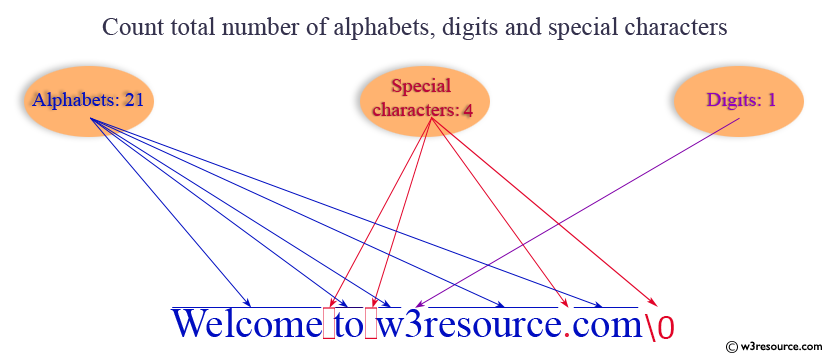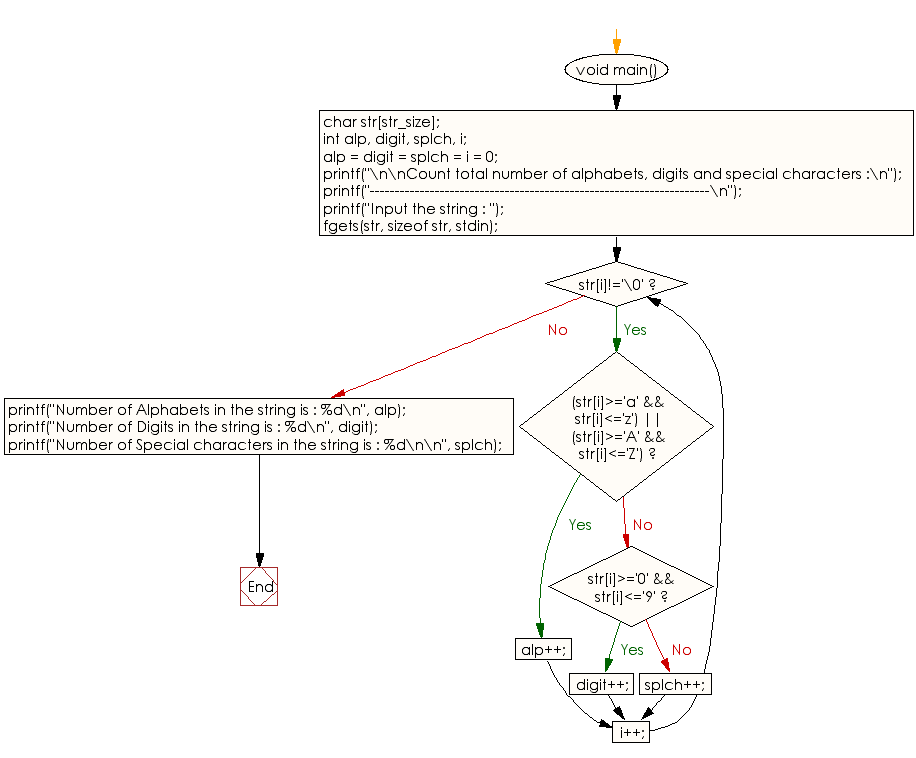﻿ C exercises: Count total number of alphabets, digits and special characters - w3resource# C Programming: Count total number of alphabets, digits and special characters

## C String: Exercise-7 with Solution

Write a program in C to count total number of alphabets, digits and special characters in a string.Sample Solution:

C Code:

``````#include <stdio.h>
#include <string.h>
#include <stdlib.h>

#define str_size 100 //Declare the maximum size of the string

void main()
{
char str[str_size];
int alp, digit, splch, i;
alp = digit = splch = i = 0;

printf("\n\nCount total number of alphabets, digits and special characters :\n");
printf("--------------------------------------------------------------------\n");
printf("Input the string : ");
fgets(str, sizeof str, stdin);

/* Checks each character of string*/

while(str[i]!='\0')
{
if((str[i]>='a' && str[i]<='z') || (str[i]>='A' && str[i]<='Z'))
{
alp++;
}
else if(str[i]>='0' && str[i]<='9')
{
digit++;
}
else
{
splch++;
}

i++;
}

printf("Number of Alphabets in the string is : %d\n", alp);
printf("Number of Digits in the string is : %d\n", digit);
printf("Number of Special characters in the string is : %d\n\n", splch);
}
```
```

Sample Output:

```Count total number of alphabets, digits and special characters :
--------------------------------------------------------------------
Input the string : Welcome to w3resource.com
Number of Alphabets in the string is : 21
Number of Digits in the string is : 1
Number of Special characters in the string is : 4
```

Flowchart:C Programming Code Editor:

Improve this sample solution and post your code through Disqus.

What is the difficulty level of this exercise?

﻿